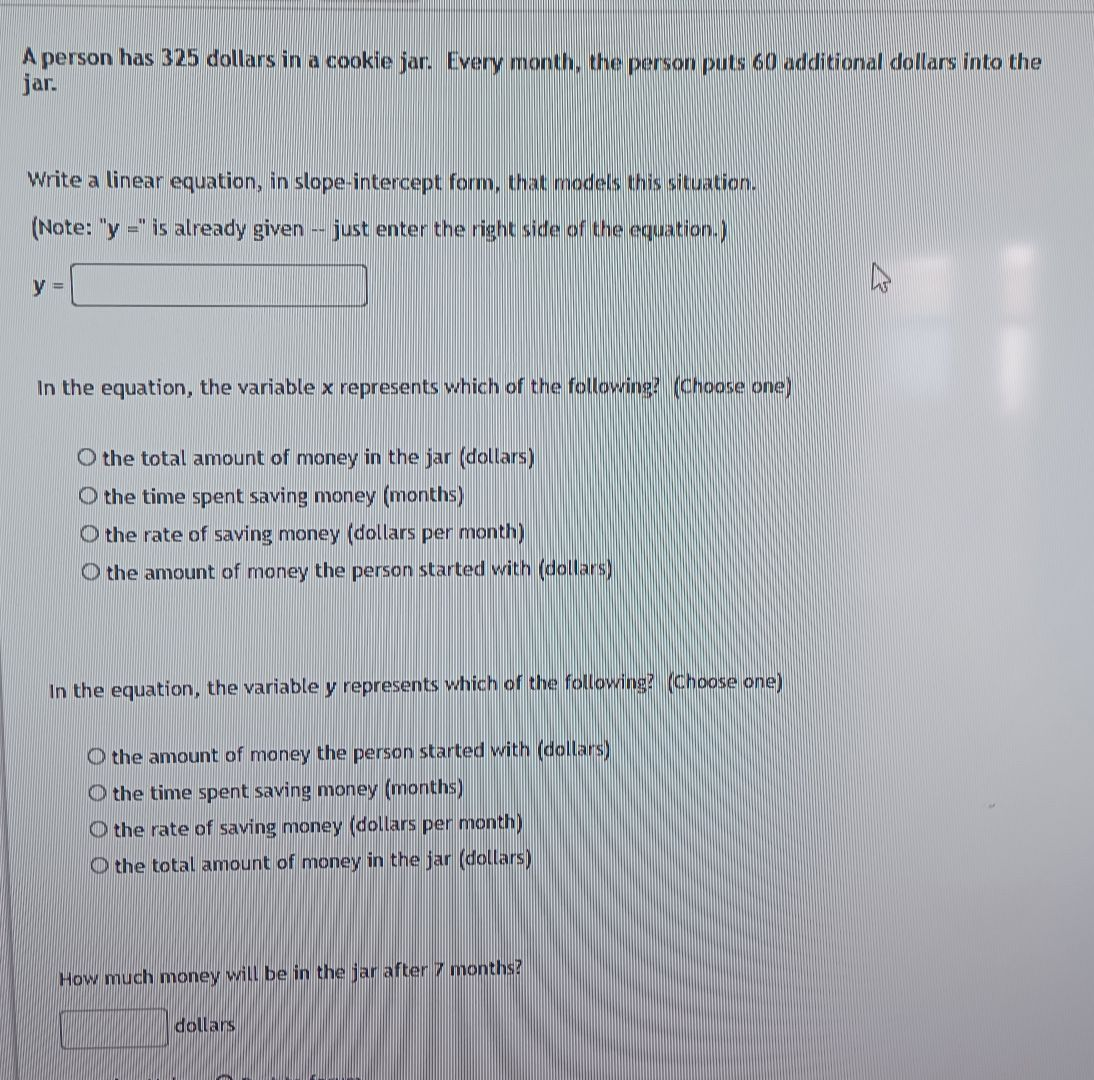### ¿Todavía tienes preguntas de matemáticas?

Pregunte a nuestros tutores expertos
Algebra
PreguntaA person has $$325$$ dollars in a cookie jar. Every month, the person puts $$60$$ additional dollars into the jar. Write a linear equation, in slope-intercept: form, that models this situation. In the equation, the variable x represents which of the following? (choose one) the total amount of money in the jar (dollars) the time spent saving money (months) the rate of saving money (dollars per month) the amount of money the person started with (dollars) In the equation, the variable $$y$$ represents which of the following? (choose one) O the amount of money the person started with (dollars) the time spent. saving money (months) the rate of saving money (dollars per month) How much money will be in the jar after $$7$$ months?